Скачать презентацию Introduction and Motivation Power consumption density has become

8f8ed9edd71fd93d5e39157d177575e2.ppt

• Количество слайдов: 17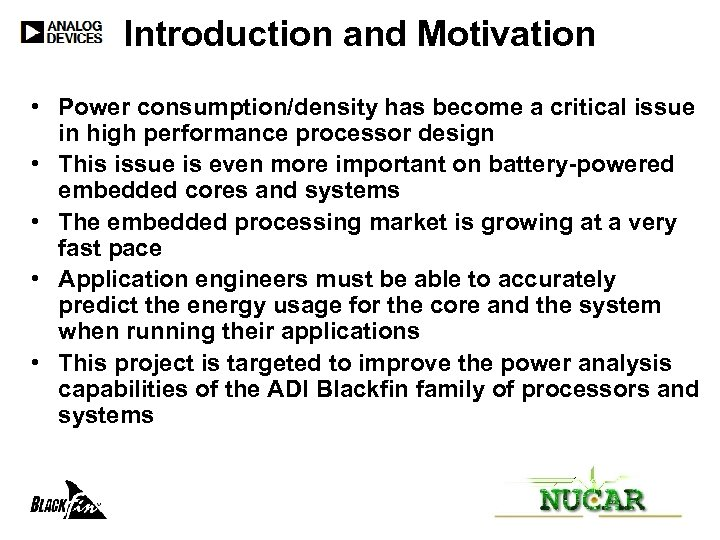Introduction and Motivation • Power consumption/density has become a critical issue in high performance processor design • This issue is even more important on battery-powered embedded cores and systems • The embedded processing market is growing at a very fast pace • Application engineers must be able to accurately predict the energy usage for the core and the system when running their applications • This project is targeted to improve the power analysis capabilities of the ADI Blackfin family of processors and systems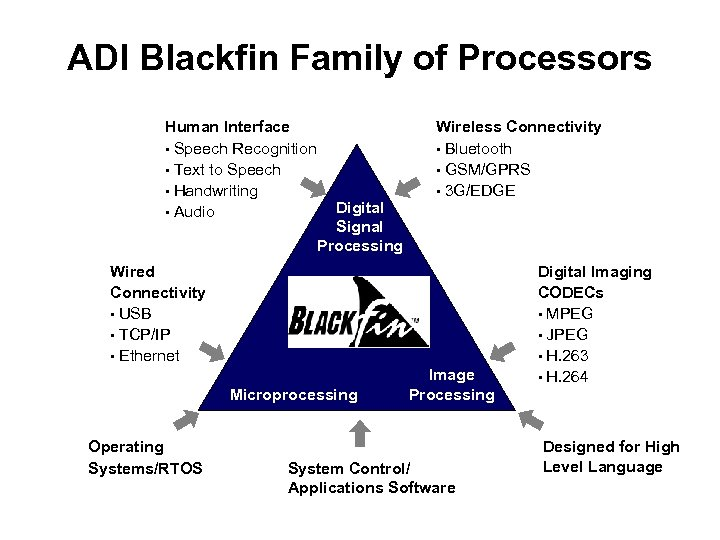ADI Blackfin Family of Processors Human Interface • Speech Recognition • Text to Speech • Handwriting • Audio Digital Signal Processing Wireless Connectivity • Bluetooth • GSM/GPRS • 3 G/EDGE Wired Connectivity • USB • TCP/IP • Ethernet Microprocessing Operating Systems/RTOS Image Processing System Control/ Applications Software Digital Imaging CODECs • MPEG • JPEG • H. 263 • H. 264 Designed for High Level Language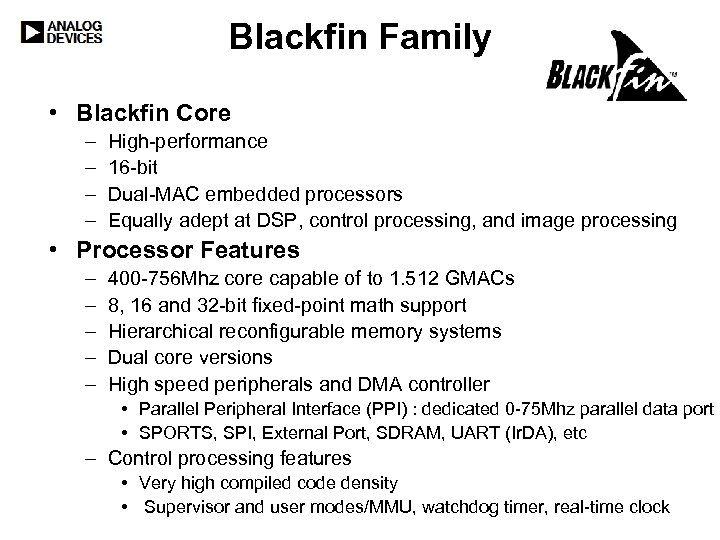Blackfin Family • Blackfin Core – – High-performance 16 -bit Dual-MAC embedded processors Equally adept at DSP, control processing, and image processing • Processor Features – – – 400 -756 Mhz core capable of to 1. 512 GMACs 8, 16 and 32 -bit fixed-point math support Hierarchical reconfigurable memory systems Dual core versions High speed peripherals and DMA controller • Parallel Peripheral Interface (PPI) : dedicated 0 -75 Mhz parallel data port • SPORTS, SPI, External Port, SDRAM, UART (Ir. DA), etc – Control processing features • Very high compiled code density • Supervisor and user modes/MMU, watchdog timer, real-time clock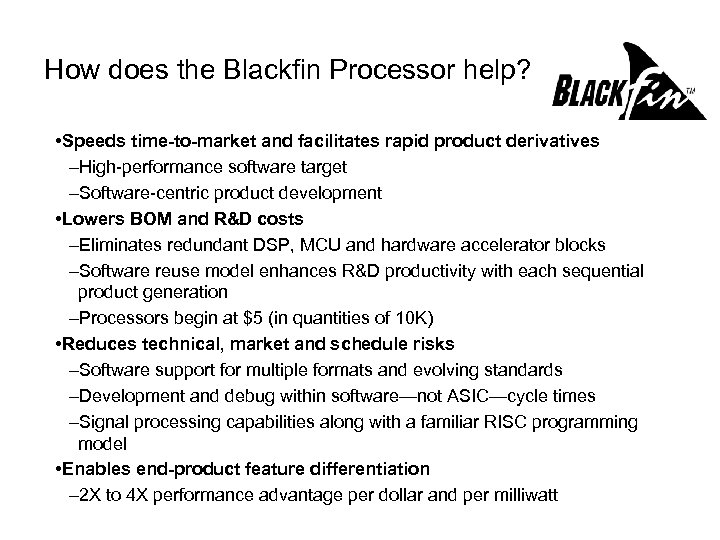How does the Blackfin Processor help? • Speeds time-to-market and facilitates rapid product derivatives –High-performance software target –Software-centric product development • Lowers BOM and R&D costs –Eliminates redundant DSP, MCU and hardware accelerator blocks –Software reuse model enhances R&D productivity with each sequential product generation –Processors begin at \$5 (in quantities of 10 K) • Reduces technical, market and schedule risks –Software support for multiple formats and evolving standards –Development and debug within software—not ASIC—cycle times –Signal processing capabilities along with a familiar RISC programming model • Enables end-product feature differentiation – 2 X to 4 X performance advantage per dollar and per milliwatt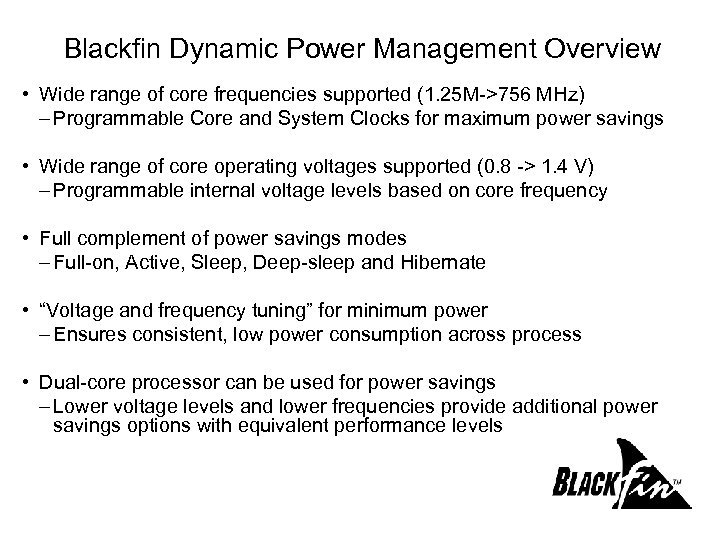Blackfin Dynamic Power Management Overview • Wide range of core frequencies supported (1. 25 M->756 MHz) – Programmable Core and System Clocks for maximum power savings • Wide range of core operating voltages supported (0. 8 -> 1. 4 V) – Programmable internal voltage levels based on core frequency • Full complement of power savings modes – Full-on, Active, Sleep, Deep-sleep and Hibernate • “Voltage and frequency tuning” for minimum power – Ensures consistent, low power consumption across process • Dual-core processor can be used for power savings – Lower voltage levels and lower frequencies provide additional power savings options with equivalent performance levels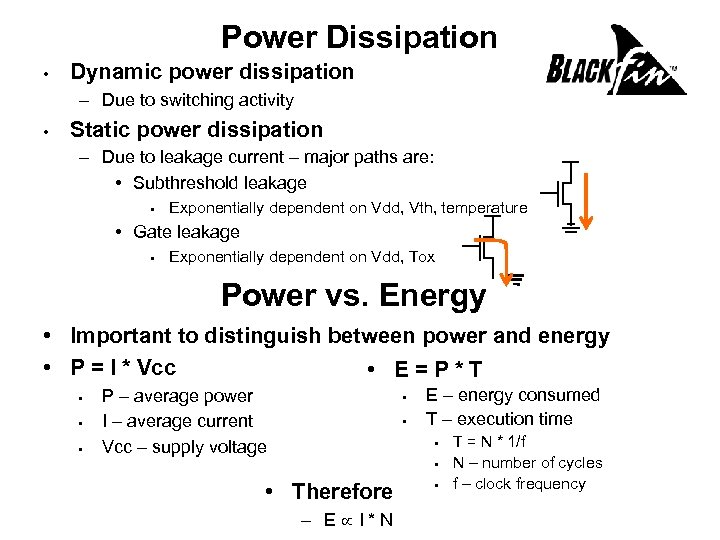Power Dissipation • Dynamic power dissipation – Due to switching activity • Static power dissipation – Due to leakage current – major paths are: • Subthreshold leakage • Exponentially dependent on Vdd, Vth, temperature • Gate leakage • Exponentially dependent on Vdd, Tox Power vs. Energy • Important to distinguish between power and energy • P = I * Vcc • E=P*T • • • P – average power I – average current Vcc – supply voltage • • E – energy consumed T – execution time • • • Therefore – E I*N • T = N * 1/f N – number of cycles f – clock frequency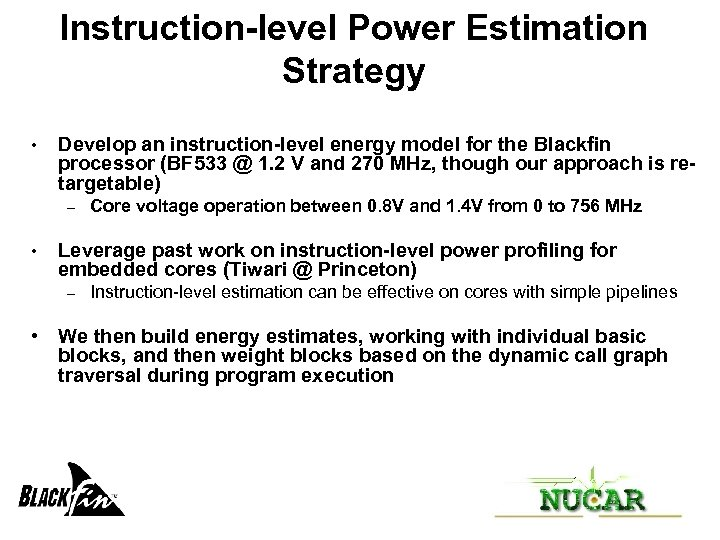Instruction-level Power Estimation Strategy • Develop an instruction-level energy model for the Blackfin processor (BF 533 @ 1. 2 V and 270 MHz, though our approach is retargetable) – • Core voltage operation between 0. 8 V and 1. 4 V from 0 to 756 MHz Leverage past work on instruction-level power profiling for embedded cores (Tiwari @ Princeton) – Instruction-level estimation can be effective on cores with simple pipelines • We then build energy estimates, working with individual basic blocks, and then weight blocks based on the dynamic call graph traversal during program execution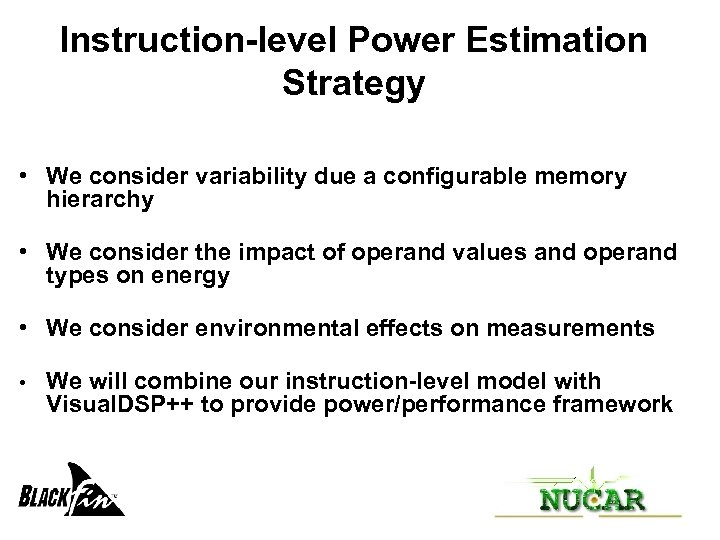Instruction-level Power Estimation Strategy • We consider variability due a configurable memory hierarchy • We consider the impact of operand values and operand types on energy • We consider environmental effects on measurements • We will combine our instruction-level model with Visual. DSP++ to provide power/performance framework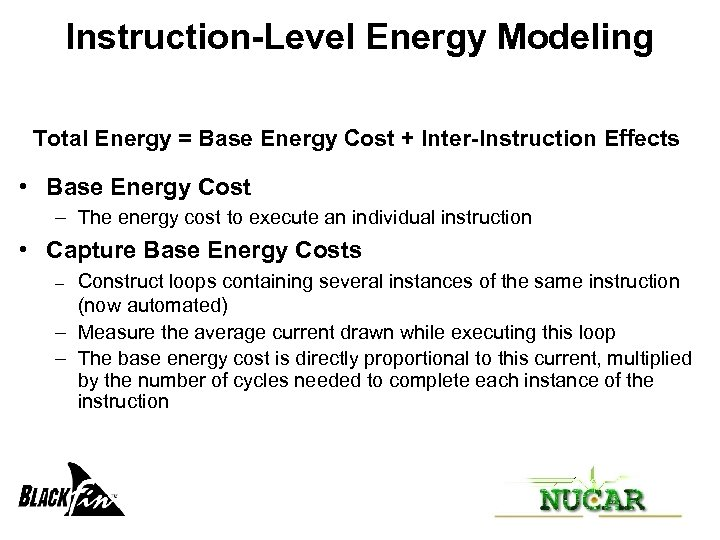Instruction-Level Energy Modeling Total Energy = Base Energy Cost + Inter-Instruction Effects • Base Energy Cost – The energy cost to execute an individual instruction • Capture Base Energy Costs Construct loops containing several instances of the same instruction (now automated) – Measure the average current drawn while executing this loop – The base energy cost is directly proportional to this current, multiplied by the number of cycles needed to complete each instance of the instruction –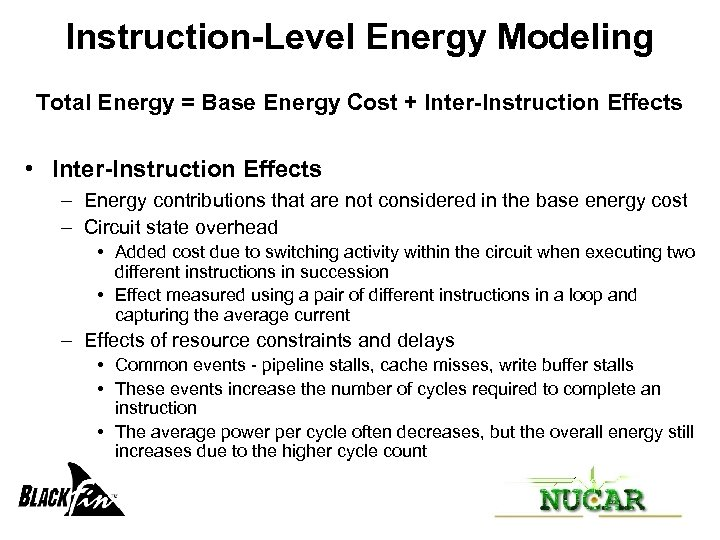Instruction-Level Energy Modeling Total Energy = Base Energy Cost + Inter-Instruction Effects • Inter-Instruction Effects – Energy contributions that are not considered in the base energy cost – Circuit state overhead • Added cost due to switching activity within the circuit when executing two different instructions in succession • Effect measured using a pair of different instructions in a loop and capturing the average current – Effects of resource constraints and delays • Common events - pipeline stalls, cache misses, write buffer stalls • These events increase the number of cycles required to complete an instruction • The average power per cycle often decreases, but the overall energy still increases due to the higher cycle count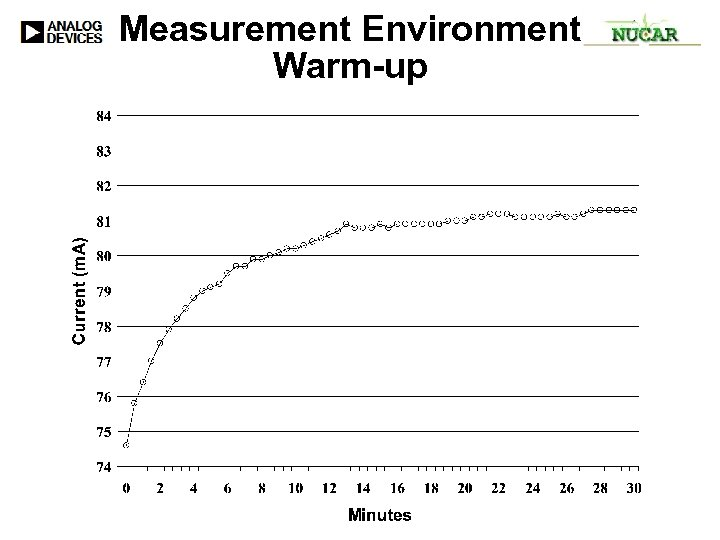Measurement Environment Warm-up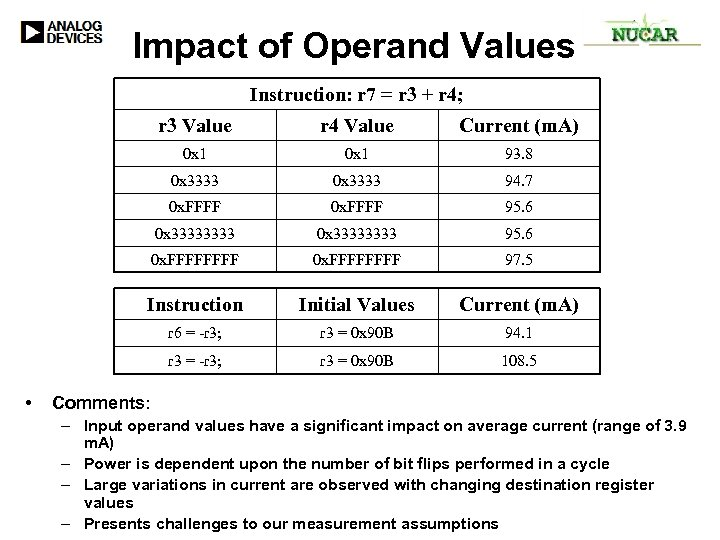Impact of Operand Values Instruction: r 7 = r 3 + r 4; r 3 Value Current (m. A) 0 x 1 93. 8 0 x 3333 94. 7 0 x. FFFF 95. 6 0 x 33333333 95. 6 0 x. FFFFFFFF 97. 5 Instruction Initial Values Current (m. A) r 6 = -r 3; r 3 = 0 x 90 B 94. 1 r 3 = -r 3; • r 4 Value r 3 = 0 x 90 B 108. 5 Comments: – Input operand values have a significant impact on average current (range of 3. 9 m. A) – Power is dependent upon the number of bit flips performed in a cycle – Large variations in current are observed with changing destination register values – Presents challenges to our measurement assumptions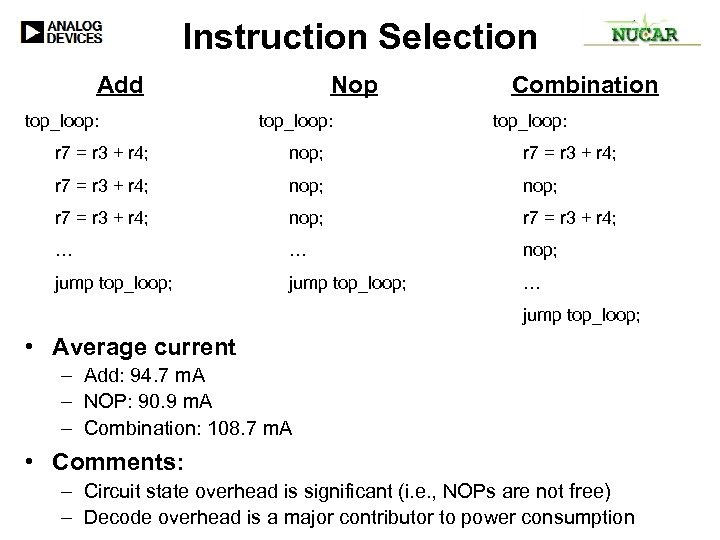Instruction Selection Add top_loop: Nop top_loop: Combination top_loop: r 7 = r 3 + r 4; nop; r 7 = r 3 + r 4; nop; r 7 = r 3 + r 4; … … nop; jump top_loop; … jump top_loop; • Average current – Add: 94. 7 m. A – NOP: 90. 9 m. A – Combination: 108. 7 m. A • Comments: – Circuit state overhead is significant (i. e. , NOPs are not free) – Decode overhead is a major contributor to power consumption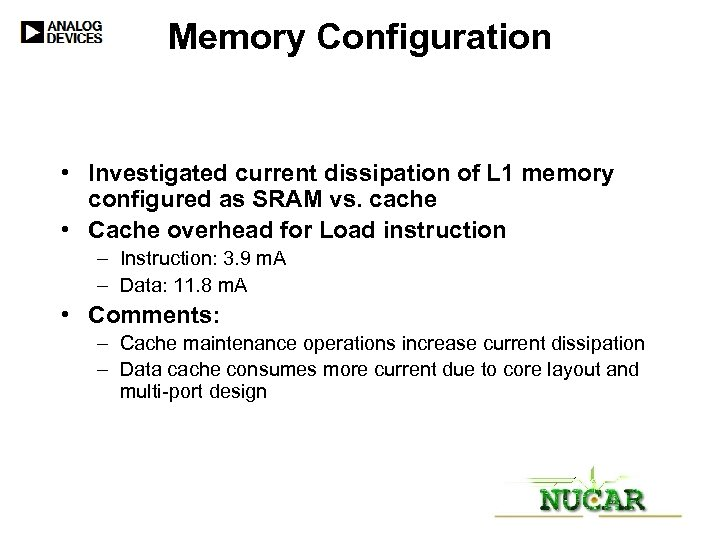Memory Configuration • Investigated current dissipation of L 1 memory configured as SRAM vs. cache • Cache overhead for Load instruction – Instruction: 3. 9 m. A – Data: 11. 8 m. A • Comments: – Cache maintenance operations increase current dissipation – Data cache consumes more current due to core layout and multi-port design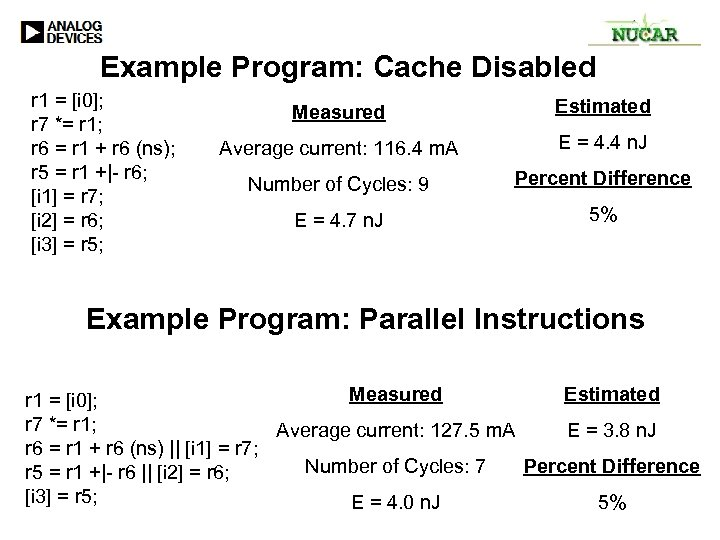Example Program: Cache Disabled r 1 = [i 0]; r 7 *= r 1; r 6 = r 1 + r 6 (ns); r 5 = r 1 +|- r 6; [i 1] = r 7; [i 2] = r 6; [i 3] = r 5; Measured Estimated Average current: 116. 4 m. A E = 4. 4 n. J Number of Cycles: 9 Percent Difference E = 4. 7 n. J 5% Example Program: Parallel Instructions Measured Estimated r 1 = [i 0]; r 7 *= r 1; Average current: 127. 5 m. A E = 3. 8 n. J r 6 = r 1 + r 6 (ns) || [i 1] = r 7; Number of Cycles: 7 Percent Difference r 5 = r 1 +|- r 6 || [i 2] = r 6; [i 3] = r 5; E = 4. 0 n. J 5%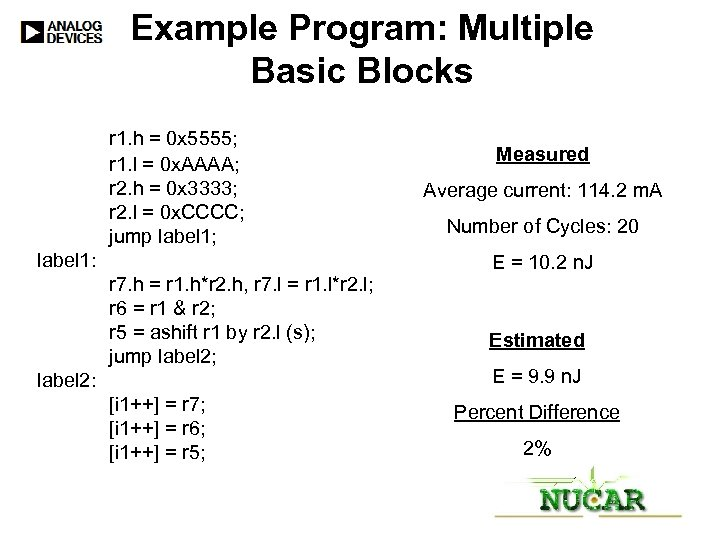Example Program: Multiple Basic Blocks r 1. h = 0 x 5555; r 1. l = 0 x. AAAA; r 2. h = 0 x 3333; r 2. l = 0 x. CCCC; jump label 1; label 1: r 7. h = r 1. h*r 2. h, r 7. l = r 1. l*r 2. l; r 6 = r 1 & r 2; r 5 = ashift r 1 by r 2. l (s); jump label 2; label 2: [i 1++] = r 7; [i 1++] = r 6; [i 1++] = r 5; Measured Average current: 114. 2 m. A Number of Cycles: 20 E = 10. 2 n. J Estimated E = 9. 9 n. J Percent Difference 2%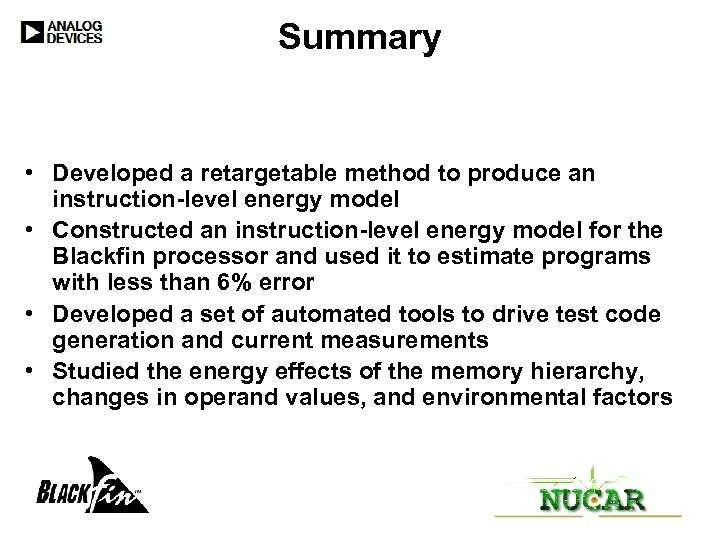Summary • Developed a retargetable method to produce an instruction-level energy model • Constructed an instruction-level energy model for the Blackfin processor and used it to estimate programs with less than 6% error • Developed a set of automated tools to drive test code generation and current measurements • Studied the energy effects of the memory hierarchy, changes in operand values, and environmental factors